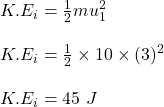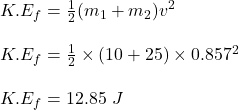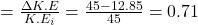## A 10-kg package drops from chute into a 25-kg cart with a velocity of 3 m/s. The cart is initially at rest and can roll freely with no frict

Question

A 10-kg package drops from chute into a 25-kg cart with a velocity of 3 m/s. The cart is initially at rest and can roll freely with no friction. Determine: a) the final velocity of the cart, b) the impulse exerted by the cart on the package, c) the fraction of the initial energy lost in the impact.

in progress 0
2 months 2021-07-31T23:51:38+00:00 1 Answers 1 views 0

(a) the final velocity of the cart is 0.857 m/s

(b) the impulse experienced by the package is 21.43 kg.m/s

(c) the fraction of the initial energy lost is 0.71

Explanation:

Given;

mass of the package, m₁ = 10 kg

mass of the cart, m₂ = 25 kg

initial velocity of the package, u₁ = 3 m/s

initial velocity of the cart, u₂ = 0

let the final velocity of the cart = v

(a) Apply the principle of conservation of linear momentum to determine common final velocity for ineleastic collision;

m₁u₁  + m₂u₂ = v(m₁  +  m₂)

10 x 3   + 25 x 0   = v(10  +  25)

30  = 35v

v = 30 / 35

v = 0.857 m/s

(b) the impulse experienced by the package;

The impulse = change in momentum of the package

J = ΔP = m₁v – m₁u₁

J = m₁(v – u₁)

J = 10(0.857 – 3)

J = -21.43 kg.m/s

the magnitude of the impulse experienced by the package = 21.43 kg.m/s

(c)

the initial kinetic energy of the package is calculated as;the final kinetic energy of the package;the fraction of the initial energy lost;